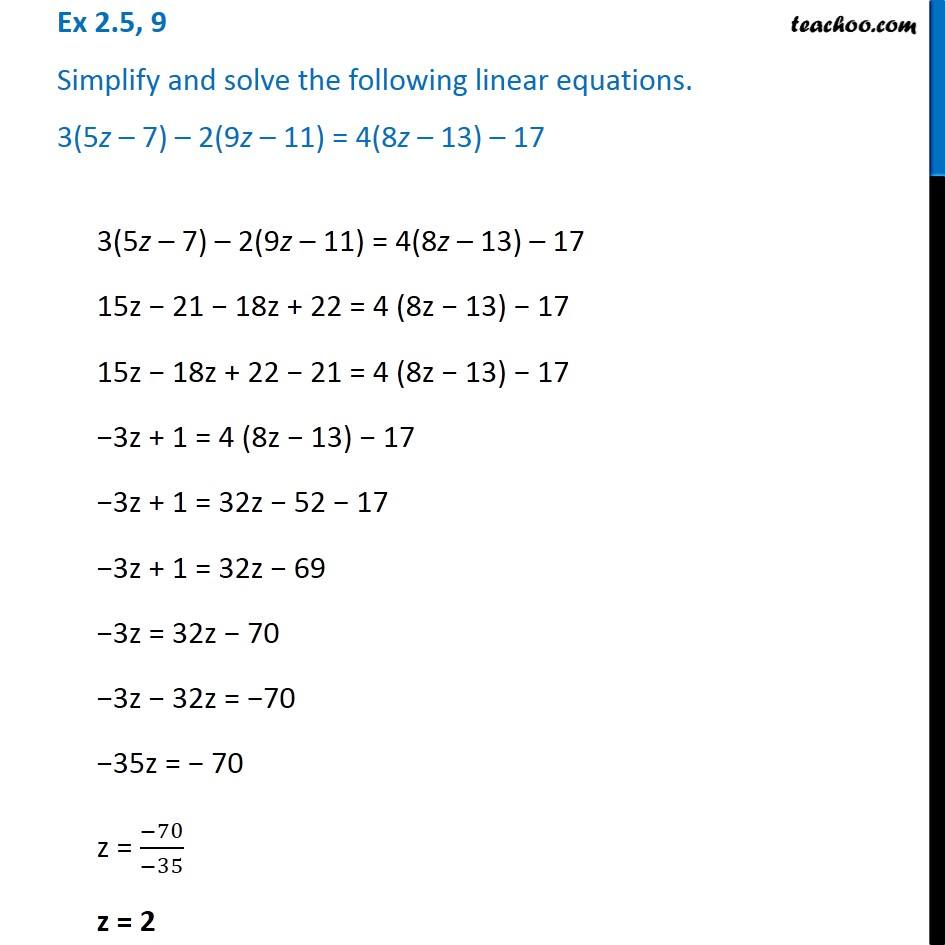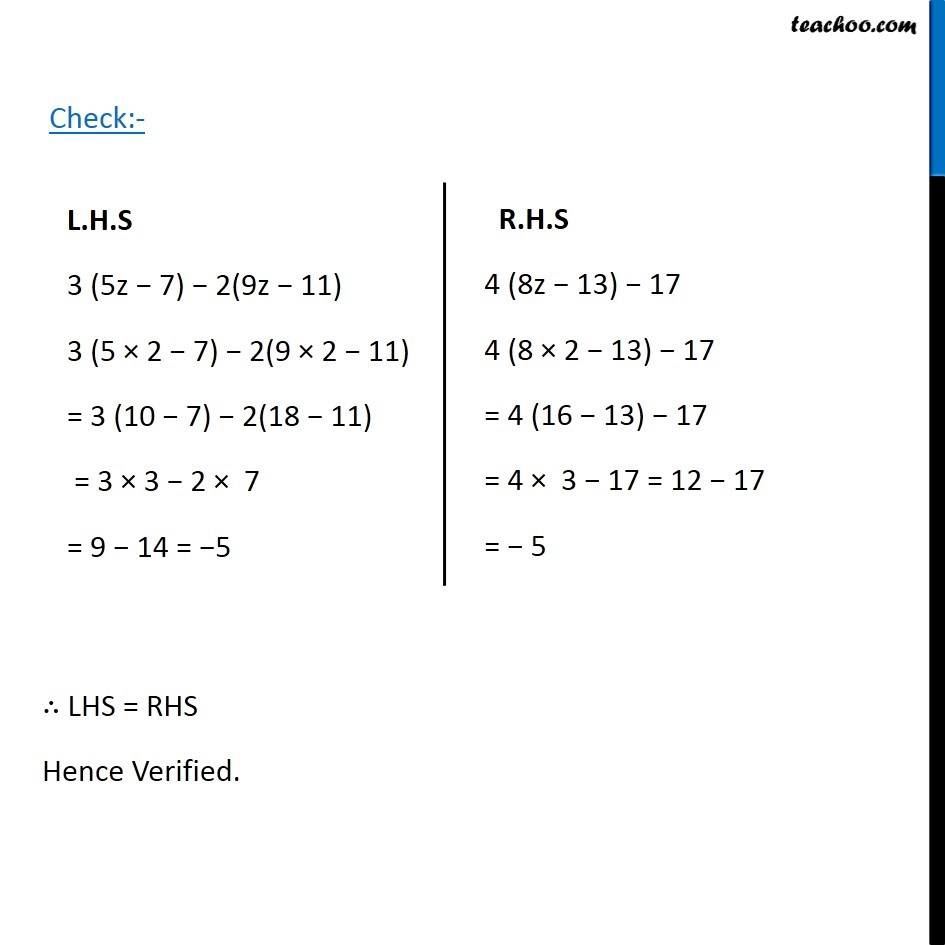1. Chapter 2 Class 8 Linear Equations in One Variable
2. Serial order wise
3. Ex 2.5

Transcript

Ex 2.5, 9 Simplify and solve the following linear equations. 3(5z 7) 2(9z 11) = 4(8z 13) 173(5z 7) 2(9z 11) = 4(8z 13) 17 15z 21 18z + 22 = 4 (8z 13) 17 15z 18z + 22 21 = 4 (8z 13) 17 3z + 1 = 4 (8z 13) 17 3z + 1 = 32z 52 17 3z + 1 = 32z 69 3z = 32z 70 3z 32z = 70 35z = 70 z = ( 70)/( 35) z = 2 L.H.S 3 (5z 7) 2(9z 11) 3 (5 2 7) 2(9 2 11) = 3 (10 7) 2(18 11) = 3 3 2 7 = 9 14 = 5 R.H.S 4 (8z 13) 17 4 (8 2 13) 17 = 4 (16 13) 17 = 4 3 17 = 12 17 = 5 LHS = RHS Hence Verified.

Ex 2.5